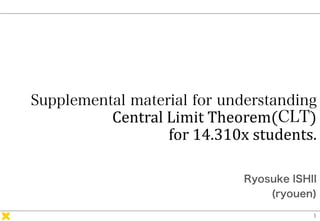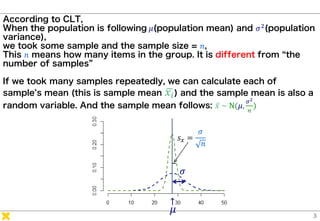Successfully reported this slideshow.×

# MITx_14310_CLT

This slide is about Central Limit Theorem(CLT) in statistics.

CLT is super useful but it is not so easy to understand, or capture the concept.
This material is those who wondering how we can understand CLT. Also this material would cover how we can think statistically; those who are used to math function sometimes wonder because the way of statistically thinking is different from general math function.

This slide is about Central Limit Theorem(CLT) in statistics.

CLT is super useful but it is not so easy to understand, or capture the concept.
This material is those who wondering how we can understand CLT. Also this material would cover how we can think statistically; those who are used to math function sometimes wonder because the way of statistically thinking is different from general math function.

### MITx_14310_CLT

1. 1. Central Limit Theorem( ) for 14.310x students. Ryosuke ISHII (ryouen)
2. 2. About author • Ryosuke ISHII (call me ryo / ryouen) • From Tokyo, Japan • Graduated from The University of Tokyo. • Current: Researcher, Grad School of System Design and Management, Keio Univ. • Enjoining MITx 14.310x and learn from a lot. • Also MITx 14.100x (Microeconomics) and HarvardX PHP525.x (Statistics) on edX.
3. 3. According to CLT, When the population is following 𝜇(population mean) and 𝜎2(population variance), we took some sample and the sample size = 𝑛, This 𝑛 means how many items in the group. It is different from “the number of samples” If we took many samples repeatedly, we can calculate each of sample’s mean (this is sample mean ഥ𝑥𝑖) and the sample mean is also a random variable. And the sample mean follows: ҧ𝑥 ~ N(𝜇, 𝜎2 𝑛 ) ↑ 𝜎 𝜇 𝑠 𝑥 = 𝜎 𝑛
4. 4. Sample size is different from the number of samples. If we compare 10 males and 15 females The sample size of the male group is 10. The sample size of the female group is 15. The number of samples (or the number of groups) is 2. The number of samples and the sample size can potentially be confusing. Sample size is the number of items within a group. Number of samples is the number of groups.” *Metin Çakanyıldırım, Computing the Standard Deviation of Sample Means
5. 5. (if you wish, you can simulate with the R code below) x <- rnorm(3300, mean=27.6,sd=sqrt(28.3)) n=10 #sample size N=1000 #the number of trials set.seed(1) ys <- vector("numeric",N) ysmean <- vector("numeric",N) ysvar <- vector("numeric",N) yssd <- vector("numeric",N) yalldata <- vector("numeric",0) for(i in 1:N){ ys <- sample(x, n) ysmean[i] = mean(ys,na.rm = TRUE) ysvar[i]= var(ys,na.rm = TRUE) yssd[i] = sd(ys,na.rm = TRUE) yalldata = c(yalldata,ys) }
6. 6. In order to understand deeper, this time assume that we know the TRUE population parameter N(𝜇, 𝜎2 ). TRUE Parameter mean 𝜇 = 27.6 variation 𝜎2 = 28.3 SD 𝜎 = 5.31 (This number is only for example) ↑ 𝜎 𝜇 Set up
7. 7. From a population following N 𝜇, 𝜎2 𝑛 = 10 Let us try sampling the first time! And we set the sample size n=10 𝑥1 34 31 25 28 26 NA 25 20 27 25 ②
8. 8. 𝜇 𝜎 We repeat it 6 times. It means we have 6 groups of samples and the sample size of each group is 10
9. 9. These 6 samples are different because each of sampling is an random sampling. But the result is not perfectly random because it is taken from a population distribution. So, we can say ”data is a representation of random variable gain from sampling.”* 𝑥2 = 25.4𝑥1 = 26.8 𝑥4 = 27.6𝑥3 = 27.5 𝑥6 = 26.9𝑥5 = 27.6 And also, we can calculate each of samples’ mean. You can see the sample mean is also a random variable.
10. 10. How to calculate the sample mean? Yes, we must know. 𝑥1 34 31 25 28 26 NA 25 20 27 25 𝑥1 =26.8 𝑥2 20 NA 22 25 NA 24 21 29 39 23 𝑥2 =25.4 𝑥3 19 16 24 29 42 27 41 21 34 22 𝑥3 =27.5 𝑥4 24 35 24 25 28 20 26 38 28 28 𝑥4 =27.6 𝑥5 27 26 28 31 23 24 NA 34 30 26 𝑥5 =27.7 𝑥6 25 26 24 28 29 NA 28 26 21 35 𝑥6 =26.9 How do you think if we take more sample? For example, we take 200 samples, and calc sample mean.
11. 11. We can plot a histogram of𝑥1~𝑥200 There are 200 averages (of samples) and each of the average is random variable. Next, we would like to calculate the distribution’s (this histogram’s) -mean of sample means ( ҧ𝑥) -variation of sample means (𝑉𝑥) -standard deviation of sample means (𝑠 𝑥)
12. 12. We can calculate it by definition. (I used R to calculate) mean of sample means ( ҧ𝑥) ҧ𝑥 = 1 𝑛 ෍ 𝑖=1 𝑛 ഥ𝑥𝑖 = ഥ𝑥1 + ഥ𝑥2 + ⋯ + 𝑥199 + 𝑥200 200 = 27.541 variation of sample means 𝑉𝑥 = 1 𝑛 − 1 ෍ 𝑖=𝑖 𝑛 ഥ𝑥𝑖 − ҧ𝑥 2 = 𝑥1 − ҧ𝑥 2 ＋ 𝑥2 − ҧ𝑥 2 + ⋯ 𝑥200 − ҧ𝑥 2 200 − 1 = 2.595608 standard deviation of sample means 𝑠 𝑥 = 𝑉𝑥 = 2.595608 = 1.611089
13. 13. We can plot a Normal distribution using the result of the calculation on a histogram we draw before. ↑ Mean ҧ𝑥 = 27.5 𝑁 ҧ𝑥, 𝑉𝑥 = 𝑁(27.5,2.6) SD: 𝑠 𝑥 = 1.6
14. 14. Let’s compare these distributions: population and sample means ↑ Mean ҧ𝑥 = 27.5 𝑁 ҧ𝑥, 𝑉𝑥 = 𝑁(27.5,2.6) 𝑆𝐷 𝑠 𝑥 = 1.6 ↑ 𝜎 = 5.3 Population mean 𝜇 = 27.6 𝑁 𝜇, 𝜎2 = 𝑁(27.6,28.3) Remember, First of all, we have a population distribution showing left. We took randomly pick up samples 200 times and the number of items within the each trial are n=10. And we calculated each samples’ mean and the distribution of the 200 sample means is showing right.
15. 15. To compare, we can integrate these graphs. What do you realize?
16. 16. We know now… The population mean is nearly samples’ mean. The samples’ variation is smaller than population’s.
17. 17. ↑ 𝜎 𝜇 Central Limit Theorem : CLT From a distribution that have 𝝁 𝒂𝒏𝒅 𝝈 𝟐 (it must NOT be following normal) We repeatedly try to take a many samples and the sample size is n. The distribution of “means of samples” are distributed and it follows 𝑁 𝜇, 𝜎2 𝑛 ↑ 𝜇 = ҧ𝑥 𝑠 𝑥 = 𝜎 𝑛 Also, we call 𝜎 𝑛 as Standard Error of the mean ഥ𝑥𝑖 SE
18. 18. Numerically examine it! The goal is to show 𝜇 = 𝑥 and 𝑠 𝑥 = 𝜎 𝑛 ↑ ҧ𝑥 = 27.5 𝑁 ҧ𝑥, 𝑉𝑥 = 𝑁(27.5,2.6) SE＝𝑠 𝑥 = 1.61 ↑ 𝜎 = 5.3 𝜇 = 27.6 𝑁 𝜇, 𝜎2 = 𝑁(27.6,28.3) 𝜇 = 27.6 ≅ ҧ𝑥 = 27.5 𝜎 𝑛 = 𝑆𝐸 = 5.3 10 = 5.3 3.16277 = 1.68 ≅ 𝑠 𝑥(𝑆𝐸) = 1.61 Almost Same! True value we already know Theoretically calculate using true value Derived from R trial
19. 19. n=2 n=5 n=10 𝑥1 34 31 25 28 26 𝑥2 20 NA 22 25 NA ⋮ 19 16 24 29 42 𝑥1000 24 35 24 25 28 𝑥1 34 31 𝑥2 20 NA ⋮ 27 26 𝑥1000 25 26 n is here If we change sample size n (and fix the number of trial)